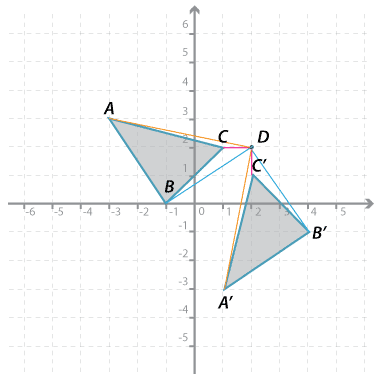#### Question 1Detailed description

Triangle $$ABC$$ is rotated around point $$D$$ by 90° in an anticlockwise direction. The image is $$A^\prime B^\prime C^\prime$$.

Give the $$x$$-coordinate and the $$y$$-coordinate of:

$$x$$ = $$y$$ = Submit answer
a $$A^\prime$$ ( , ).
b $$B^\prime$$ ( , ).
c $$C^\prime$$ ( , ).

Place answers in both checkboxes before submitting.